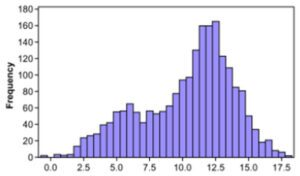# Can I estimate an SEM if the sample data are not normally distributed?Continuous distributions are typically described by their mean (central tendency), variance (spread), skew (asymmetry), and kurtosis (thickness of tails). A normal distribution assumes a skew and kurtosis of zero, but truly normal distributions are rare in practice. Unfortunately, the fitting of standard SEMs to non-normal data can result in inflated model test statistics (leading models to be rejected more often than they should) and under-estimated standard errors (leading tests of individual parameters to be accepted more often then they should be). There are a number of important issues that must be considered when addressing this in practice.

First, the assumption of normality is a characteristic of the estimator and not the model itself. So “the SEM” doesn’t assume normality, but the widely-used normal-theory maximum likelihood (ML) estimator does. Second, the assumption of normality applies to the residuals and is thus only relevant for dependent variables as defined in a given model; in contrast, the independent variables can take any distributional form at all (e.g., binary, count, bi-modal, long tail, etc.). Third, there are no well-defined numerical cut-offs for skew or kurtosis to determine whether a sample distribution is sufficiently non-normal to introduce problems in estimation, and tests of multivariate skew and kurtosis tend to be over-powered (significant even when the departure from normality is too slight to matter). Similarly, because the assumption of normality is on the residuals, the overall distributions of the observed variables are only indirectly indicative of the residual distributions. Nevertheless, in practice, we tend to examine histograms and scatter plots of the dependent variables to make a (somewhat subjective) determination of whether univariate and bivariate normality appear to be approximately satisfied.

If normality is in doubt, remedial steps can be taken to help mitigate problems associated with violating this assumption. One option is to apply non-linear transformations to the problem variables (e.g., natural log, square root). Although these can sometimes help sample data better approximate a normal distribution, nonlinear transformations also alter the relationships between variables (e.g., a linear relationship becomes nonlinear under transformation) and can impede substantive interpretation. A second and often better option is to use a method of estimation that is less impacted by the deleterious effects of non-normality like robust maximum likelihood (widely available, with some variation, in many software packages). The underlying mechanics of robust ML are complex, but it functionally introduces data-based corrections to the test statistic and standard errors to offset the bias introduced by the non-normal distribution. Simulation studies have shown that these robust estimators work exceedingly well under conditions commonly encountered in applied research and robust methods are often the best option available, and this is what we generally recommend in practice.

However, all of the above assumes that the distributions remain continuous. If the dependent variable distribution is discrete (e.g., binary, ordinal, count) then a more complex non-linear model is needed. We will discuss these options in a future help desk topic.

Curran, P. J., West, S. G., & Finch, J. F. (1996). The robustness of test statistics to nonnormality and specification error in confirmatory factor analysis. Psychological Methods, 1, 16-29.

Finney, S. J., & DiStefano, C. (2006). Non-normal and categorical data in structural equation modeling. In G. Hancock & R. Mueller (Eds.), Structural Equation Modeling: A Second Course, 269-314. Greenwich, CT: Information Age Publishing.

Satorra, A., & Bentler, P. M. (1994). Corrections to test statistics and standard errors in covariance structure analysis. In A. von Eye & C. C. Clogg (Eds.), Latent variables analysis: Applications for developmental research (pp. 399-419). Thousand Oaks, CA, US: Sage Publications, Inc.

West, S.G., Finch, J.F., & Curran, P.J. (1995). Structural equation models with non-normal variables: Problems and remedies. In R. Hoyle (Ed.), Structural Equation Modeling: Concepts, Issues and Applications, (pp. 56-75). Newbury Park, CA: Sage.

## What’s the best way to determine the number of latent classes in a finite mixture analysis?

Selecting the number of classes (or components) is one of the most challenging decisions to make when fitting a finite mixture model (including latent class analysis and latent profile analysis). In this post, we talk through the conventional wisdom on class enumeration, as well as when this breaks down.

## I’m reporting within- and between-group effects in from a multilevel model, and my reviewer says I need to address “sampling error” in the group means. What does this mean, and what can I do to address this?

Why between-group effects estimating in MLMs are sometimes biased, and what to do about it

## I fit a multilevel model and got the warning message “G Matrix is Non-Positive Definite.” What does this mean and what should I do about it?

Received the cryptic warning message “G matrix is non-positive definite”? Learn what this means and what to do about it.

## My advisor told me I should group-mean center my predictors in my multilevel model because it might “make my effects significant” but this doesn’t seem right to me. What exactly is involved in centering predictors within the multilevel model?

How to specify multilevel models to obtain within- and between-group effects through centering lower-level predictors.

## What are ROC curves and how are these used to aid decision making?

ROC curves are used to identify cut points on continuous measures at which to make binary decisions. An example is a depression screener used to identify people who may qualify for a major depression diagnosis. This post explains the concepts behind ROC curves and how they are used for decision making.